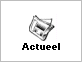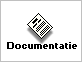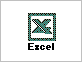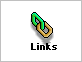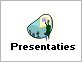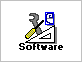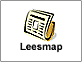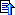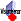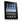## 7. Inverse van a (mod b)

```Function Inverse(a, b)
s = 0
old_s = 1
t = 1
old_t = 1
r = b
old_r = a
While r <> 0
q = old_r \ r
p = r
r = old_r - q * p
old_r = p
p = s
s = old_s - q * p
old_s = p
p = t
t = old_t - q * p
old_t = p
Wend
If old_s < 0 Then
Inverse = old_s + b
Else
Inverse = old_s
End If
End Function```
https://en.wikipedia.org/wiki/Extended_GCD